# Machine Learning Lecture 2 Concept Learning and Version

• Slides: 20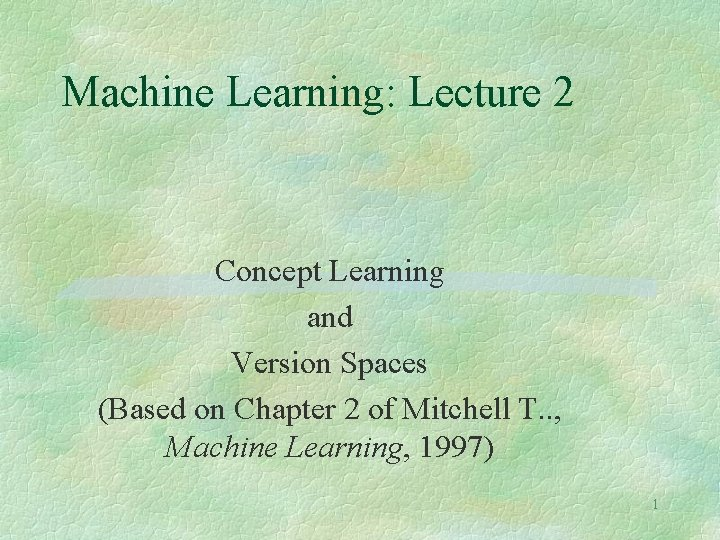Machine Learning: Lecture 2 Concept Learning and Version Spaces (Based on Chapter 2 of Mitchell T. . , Machine Learning, 1997) 1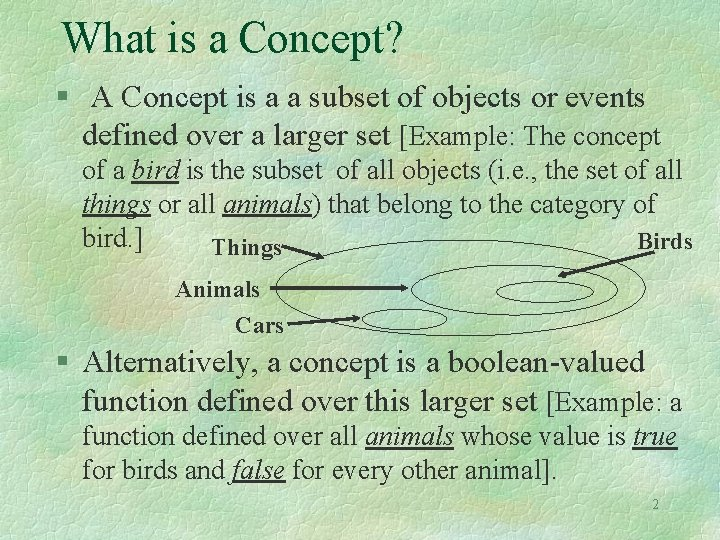What is a Concept? § A Concept is a a subset of objects or events defined over a larger set [Example: The concept of a bird is the subset of all objects (i. e. , the set of all things or all animals) that belong to the category of bird. ] Birds Things Animals Cars § Alternatively, a concept is a boolean-valued function defined over this larger set [Example: a function defined over all animals whose value is true for birds and false for every other animal]. 2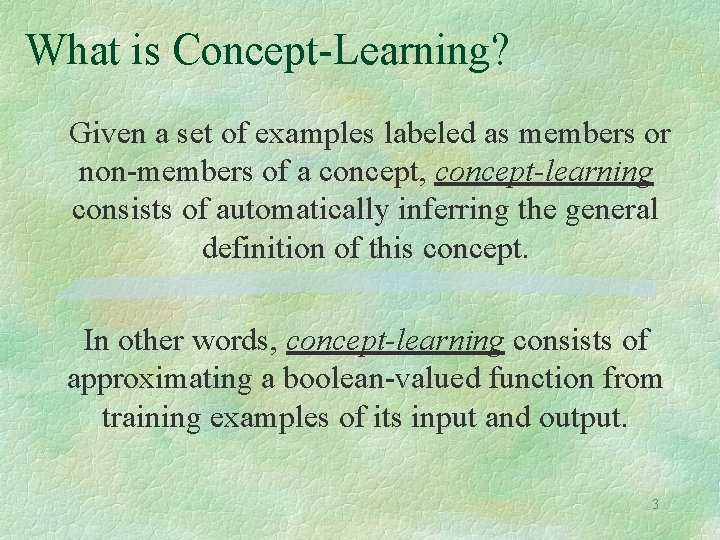What is Concept-Learning? Given a set of examples labeled as members or non-members of a concept, concept-learning consists of automatically inferring the general definition of this concept. In other words, concept-learning consists of approximating a boolean-valued function from training examples of its input and output. 3Example of a Concept Learning task § Concept: Good Days for Water Sports (values: Yes, No) § Attributes/Features: l l l Sky (values: Sunny, Cloudy, Rainy) Air. Temp (values: Warm, Cold) Humidity (values: Normal, High) Wind (values: Strong, Weak) Water (Warm, Cool) Forecast (values: Same, Change) class § Example of a Training Point: <Sunny, Warm, High, Strong, Warm, Same, Yes> 4Example of a Concept Learning task Database: Day 1 2 3 4 Sky Air. Temp Humidity Wind Water Forecast Water. Sport Sunny Warm Normal Strong Warm Same Yes Sunny Warm High Strong Warm Same Yes Rainy Cold High Strong Warm Change No Sunny Warm High Strong Cool Change Yes class Chosen Hypothesis Representation: Conjunction of constraints on each attribute where: • “? ” means “any value is acceptable” • “ 0” means “no value is acceptable” Example of a hypothesis: <? , Cold, High, ? , ? > (If the air temperature is cold and the humidity high then it is a good day for water sports) 5Example of a Concept Learning task § Goal: To infer the “best” concept-description from the set of all possible hypotheses (“best” means “which best generalizes to all (known or unknown) elements of the instance space”. . concept-learning is an ill-defined task) § Most General Hypothesis: Everyday is a good day for water sports <? , ? , ? , ? > § Most Specific Hypothesis: No day is a good day for water sports <0, 0, 0, 0> 6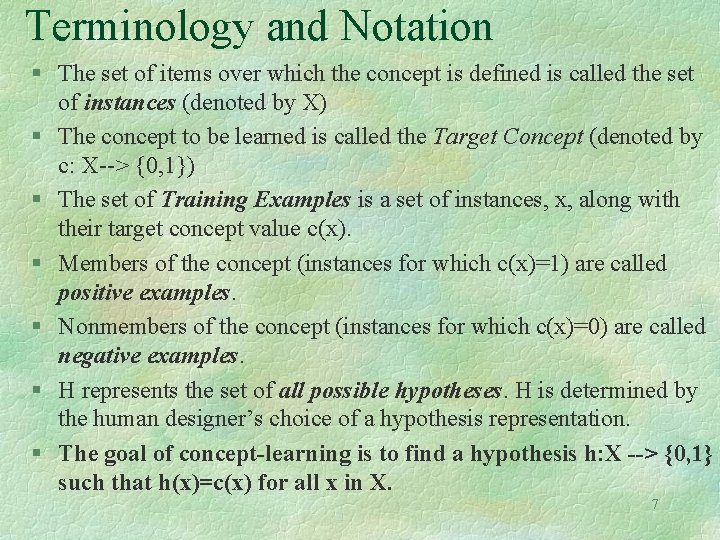Terminology and Notation § The set of items over which the concept is defined is called the set of instances (denoted by X) § The concept to be learned is called the Target Concept (denoted by c: X--> {0, 1}) § The set of Training Examples is a set of instances, x, along with their target concept value c(x). § Members of the concept (instances for which c(x)=1) are called positive examples. § Nonmembers of the concept (instances for which c(x)=0) are called negative examples. § H represents the set of all possible hypotheses. H is determined by the human designer’s choice of a hypothesis representation. § The goal of concept-learning is to find a hypothesis h: X --> {0, 1} such that h(x)=c(x) for all x in X. 7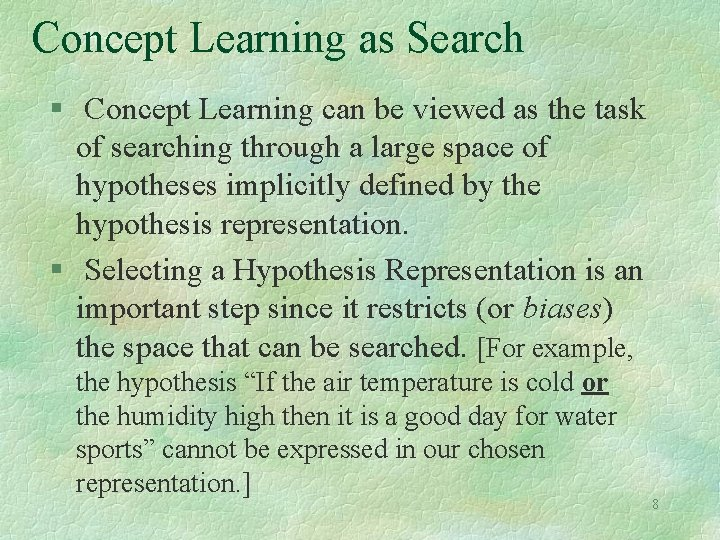Concept Learning as Search § Concept Learning can be viewed as the task of searching through a large space of hypotheses implicitly defined by the hypothesis representation. § Selecting a Hypothesis Representation is an important step since it restricts (or biases) the space that can be searched. [For example, the hypothesis “If the air temperature is cold or the humidity high then it is a good day for water sports” cannot be expressed in our chosen representation. ] 8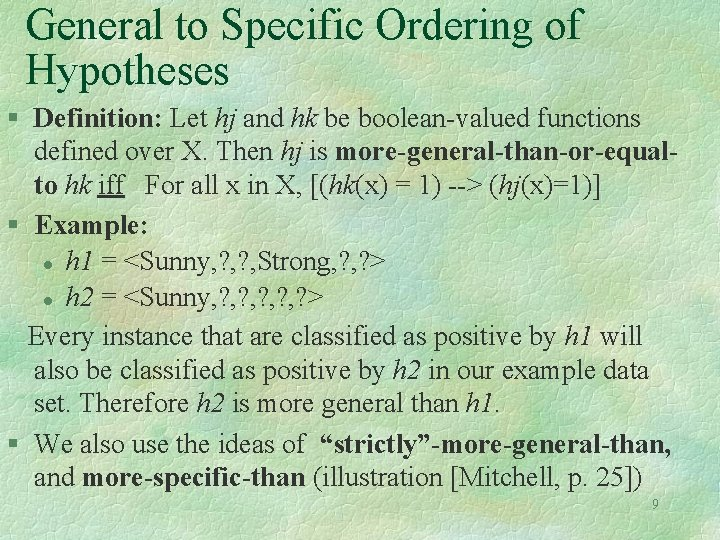General to Specific Ordering of Hypotheses § Definition: Let hj and hk be boolean-valued functions defined over X. Then hj is more-general-than-or-equalto hk iff For all x in X, [(hk(x) = 1) --> (hj(x)=1)] § Example: l h 1 = <Sunny, ? , Strong, ? > l h 2 = <Sunny, ? , ? , ? > Every instance that are classified as positive by h 1 will also be classified as positive by h 2 in our example data set. Therefore h 2 is more general than h 1. § We also use the ideas of “strictly”-more-general-than, and more-specific-than (illustration [Mitchell, p. 25]) 9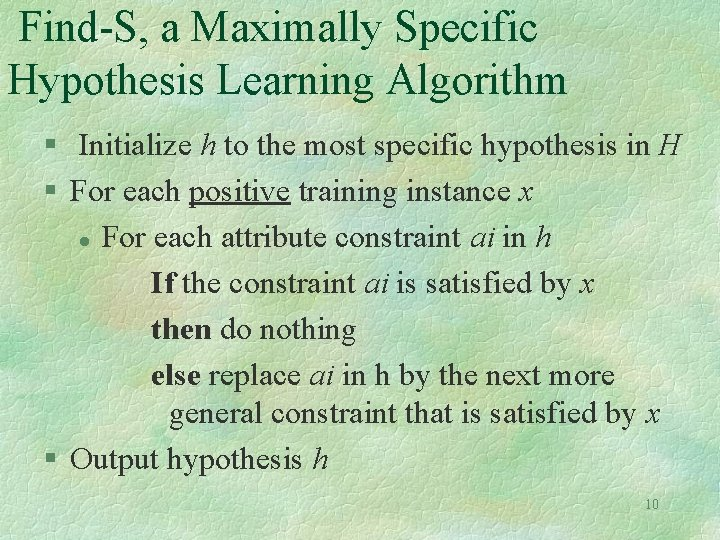Find-S, a Maximally Specific Hypothesis Learning Algorithm § Initialize h to the most specific hypothesis in H § For each positive training instance x l For each attribute constraint ai in h If the constraint ai is satisfied by x then do nothing else replace ai in h by the next more general constraint that is satisfied by x § Output hypothesis h 10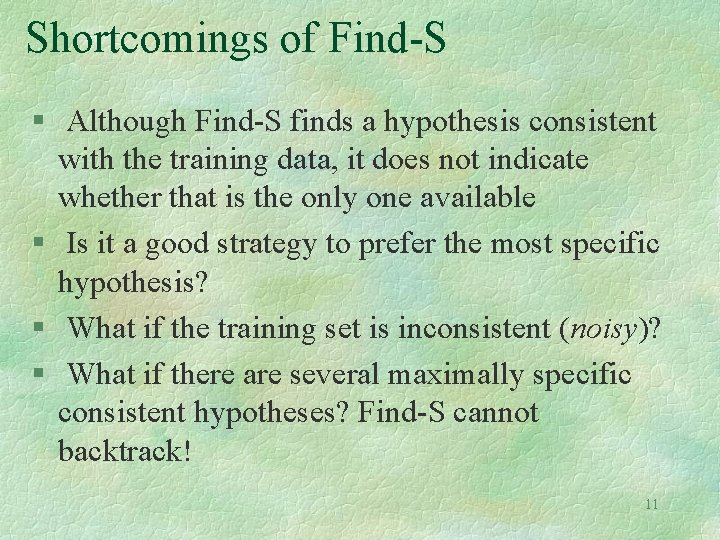Shortcomings of Find-S § Although Find-S finds a hypothesis consistent with the training data, it does not indicate whether that is the only one available § Is it a good strategy to prefer the most specific hypothesis? § What if the training set is inconsistent (noisy)? § What if there are several maximally specific consistent hypotheses? Find-S cannot backtrack! 11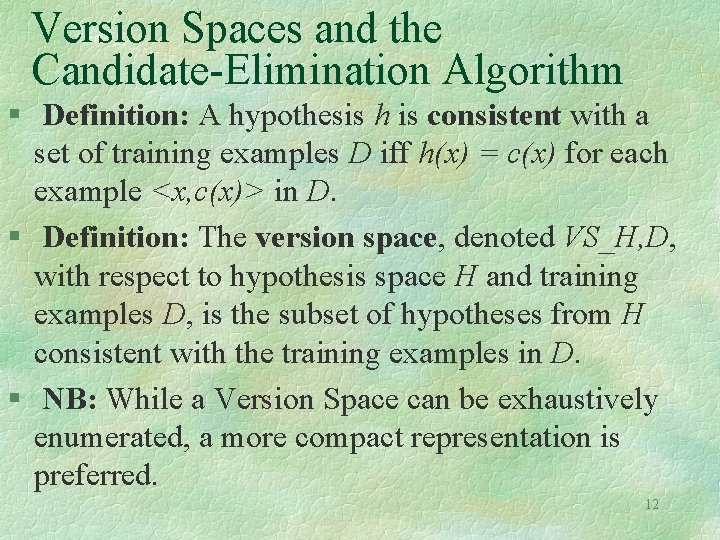Version Spaces and the Candidate-Elimination Algorithm § Definition: A hypothesis h is consistent with a set of training examples D iff h(x) = c(x) for each example <x, c(x)> in D. § Definition: The version space, denoted VS_H, D, with respect to hypothesis space H and training examples D, is the subset of hypotheses from H consistent with the training examples in D. § NB: While a Version Space can be exhaustively enumerated, a more compact representation is preferred. 12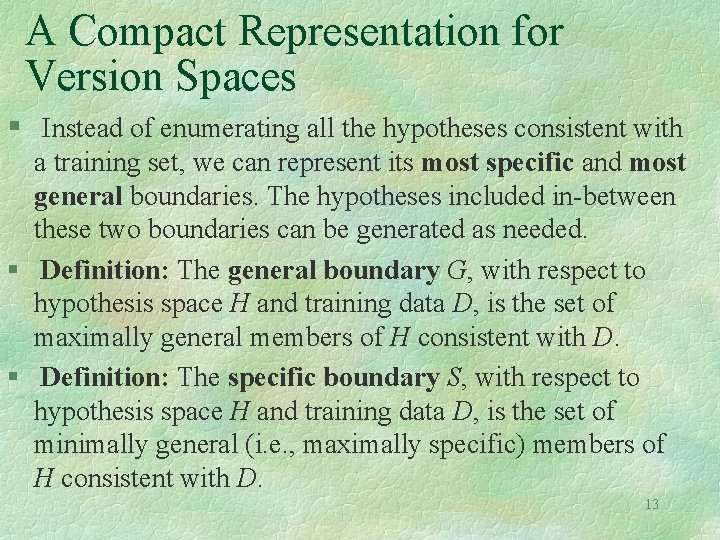A Compact Representation for Version Spaces § Instead of enumerating all the hypotheses consistent with a training set, we can represent its most specific and most general boundaries. The hypotheses included in-between these two boundaries can be generated as needed. § Definition: The general boundary G, with respect to hypothesis space H and training data D, is the set of maximally general members of H consistent with D. § Definition: The specific boundary S, with respect to hypothesis space H and training data D, is the set of minimally general (i. e. , maximally specific) members of H consistent with D. 13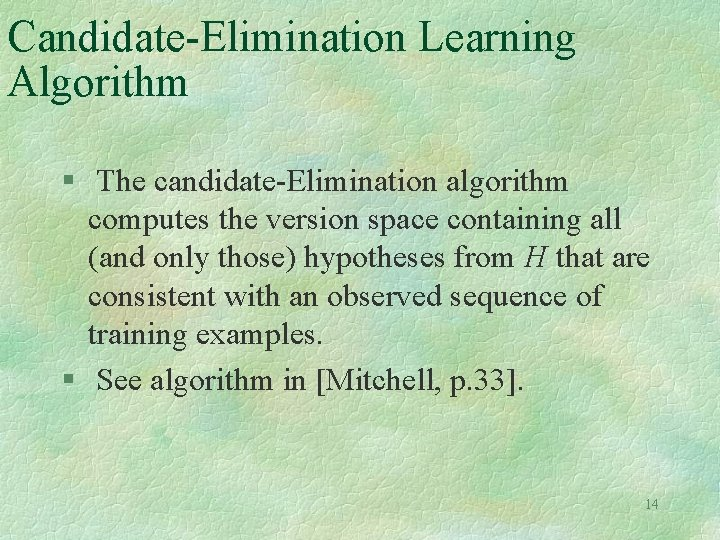Candidate-Elimination Learning Algorithm § The candidate-Elimination algorithm computes the version space containing all (and only those) hypotheses from H that are consistent with an observed sequence of training examples. § See algorithm in [Mitchell, p. 33]. 14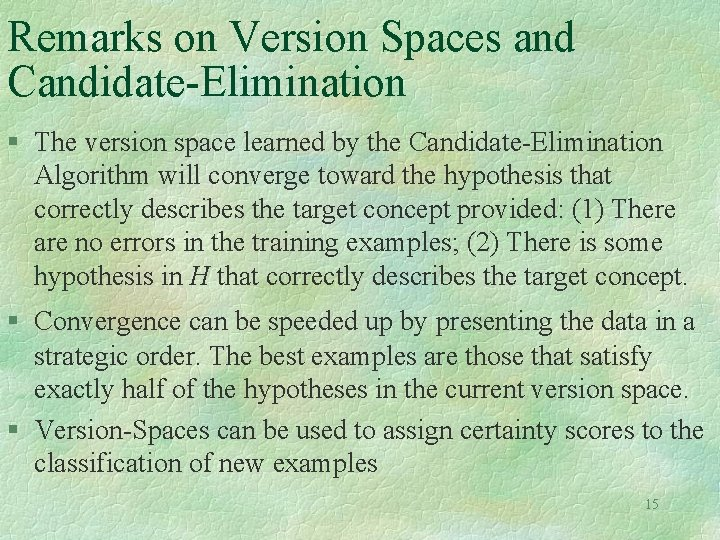Remarks on Version Spaces and Candidate-Elimination § The version space learned by the Candidate-Elimination Algorithm will converge toward the hypothesis that correctly describes the target concept provided: (1) There are no errors in the training examples; (2) There is some hypothesis in H that correctly describes the target concept. § Convergence can be speeded up by presenting the data in a strategic order. The best examples are those that satisfy exactly half of the hypotheses in the current version space. § Version-Spaces can be used to assign certainty scores to the classification of new examples 15Inductive Bias I: A Biased Hypothesis Space Database: Day 1 2 3 Sky Air. Temp Humidity Wind Water Forecast Water. Sport Sunny Warm Normal Strong Cool Change Yes Cloudy Warm Normal Strong Cool Change Yes Rainy Warm Normal Strong Cool Change No class § Given our previous choice of the hypothesis space representation, no hypothesis is consistent with the above database: we have BIASED the learner to consider only conjunctive hypotheses 16Inductive Bias II: An Unbiased Learner § In order to solve the problem caused by the bias of the hypothesis space, we can remove this bias and allow the hypotheses to represent every possible subset of instances. The previous database could then be expressed as: <Sunny, ? , ? , ? > v <Cloudy, ? , ? , ? > § However, such an unbiased learner is not able to generalize beyond the observed examples!!!! All the non-observed examples will be well-classified by half the hypotheses of the version space and misclassified by the other half. 17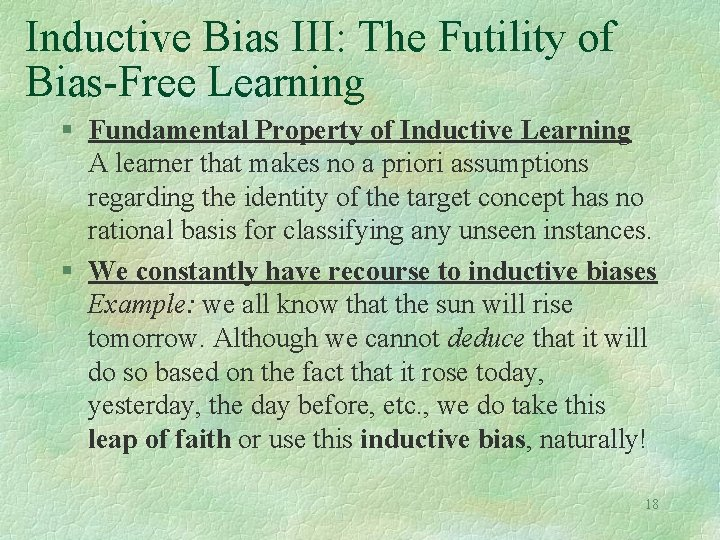Inductive Bias III: The Futility of Bias-Free Learning § Fundamental Property of Inductive Learning A learner that makes no a priori assumptions regarding the identity of the target concept has no rational basis for classifying any unseen instances. § We constantly have recourse to inductive biases Example: we all know that the sun will rise tomorrow. Although we cannot deduce that it will do so based on the fact that it rose today, yesterday, the day before, etc. , we do take this leap of faith or use this inductive bias, naturally! 18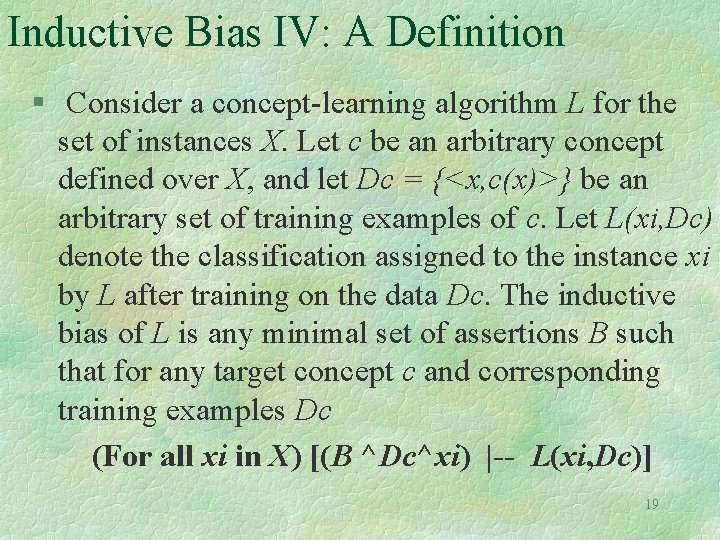Inductive Bias IV: A Definition § Consider a concept-learning algorithm L for the set of instances X. Let c be an arbitrary concept defined over X, and let Dc = {<x, c(x)>} be an arbitrary set of training examples of c. Let L(xi, Dc) denote the classification assigned to the instance xi by L after training on the data Dc. The inductive bias of L is any minimal set of assertions B such that for any target concept c and corresponding training examples Dc (For all xi in X) [(B ^Dc^xi) |-- L(xi, Dc)] 19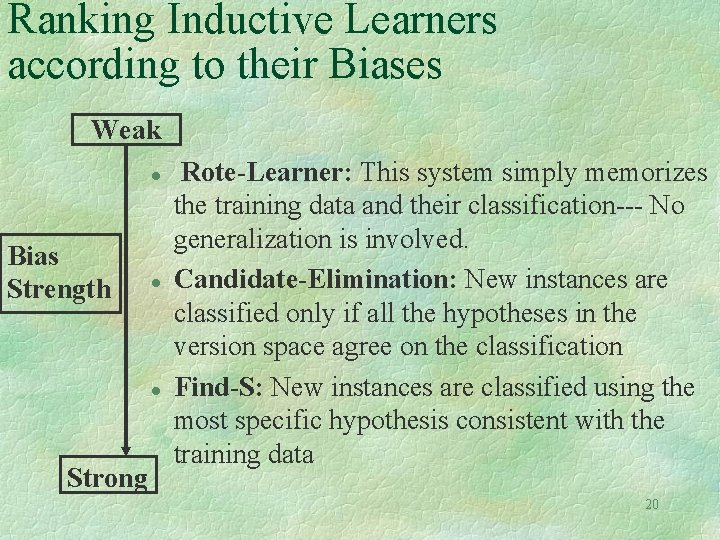Ranking Inductive Learners according to their Biases Weak l Bias Strength l l Strong Rote-Learner: This system simply memorizes the training data and their classification--- No generalization is involved. Candidate-Elimination: New instances are classified only if all the hypotheses in the version space agree on the classification Find-S: New instances are classified using the most specific hypothesis consistent with the training data 20